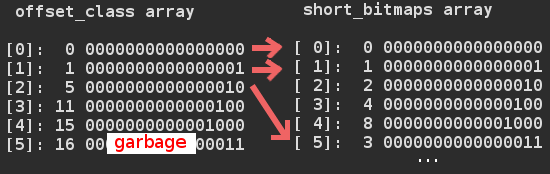# How do succinct data types work in libcds? – Part II

## How does RRR work?

todo

#### Building the RRR structure

When you build an RRR structure you have to define the bitstring and a sample rate.

`BitSequenceRRR::BitSequenceRRR(const BitString & bs, uint sample_rate);`

bs is the source data
sample_rate is todo

BLOCK_SIZE := 15, by definition.
C_field_bits := 4, it means how many bits required to express BLOCK_SIZE

Note: I use these values from here without the variable names.

C_len := number of 4 bit blocks in source data
C := uint array with size enough to hold C_len * 4 bits

It makes a table_offset object named “E” with BLOCK_SIZE parameter:

`table_offset::table_offset(uint u)`

This call creates:

• 2 simple arrays: short_bitmaps[2^(15+1)], offset_class[15+2];
• 2 two dimensional arrays (with dimension (15+1)x(15+1)): binomial, log2binomial
• 1 array (_List) with size 2^(15+1+1)

See how these tables look like: binomial table, log2binomial table, short_bitmaps, offset_class
You get the log2binomial table by ceiling the log2 values of the binomial table.
You get short_bitmaps array by iterating all possible numbers with the given number of 1s in it’s binary form.
offset_class array contains the offsets (in short_bitmaps) of the classes of ones.Note: short_bitmaps and offset_class tables was generated with BLOCK_SIZE set to 4 for readability.

I have no idea what is the purpose of _List after the creation of these tables (it’s saved as rev_offset). The algorithm for filling sort_bitmaps and offset_class arrays are REALLY complicated, contains deep recursion, names written in Spanish.. so I skip describing it’s inner working.

todo

It iterates through the source data and counts the number of ones in 15 bit groups (see BLOCK_SIZE). In the iteration it always sets the next 4 bits (see C_field_bits) of array “C” with this number.

todo

While iterating the total number of ones is summed and … todo

Getting the 15 bit parts and setting the 4 bit parts in uint arrays is done by using the uint arrays as packed arrays.

Note:
These are the functions for handling arrays as packed arrays (libcdsBasics.h):

```inline uint get_var_field(const uint *A, const size_t ini, const size_t fin);
inline void set_field(uint *A, const size_t len, const size_t index, const uint x)
```

todo

#### Problems and inefficiencies in RRR

• Zeroing is done one by one. A standard library call should be used instead!
• generaClase function uses deep recursion which is inefficient
• There are lots of +1s,+2s in array dimensions which are unnecessary
• todo

## 2 thoughts on “How do succinct data types work in libcds? – Part II”

1.András Majdán Post author

At that time I’ve lost interest (and time) for working out how RRR works in libcds. Visit Alex Bowe’s page if you are interested in RRR: https://alexbowe.com/rrr/

This site uses Akismet to reduce spam. Learn how your comment data is processed.